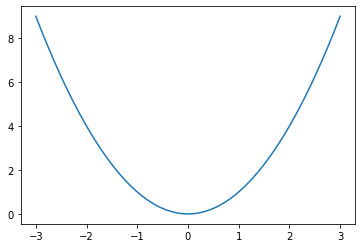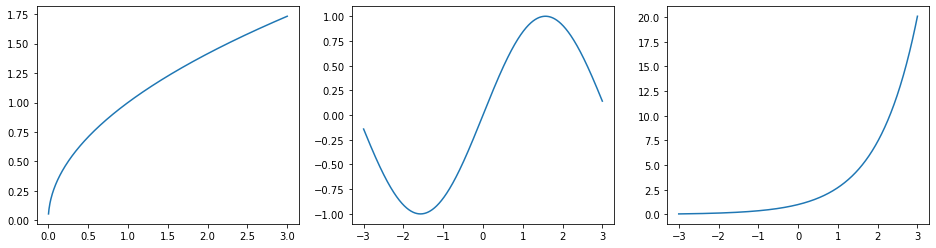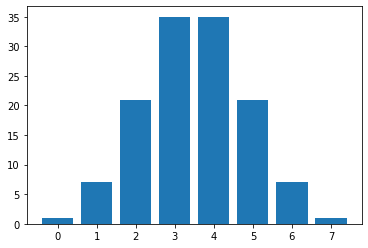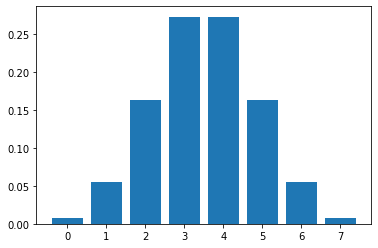Courses

Explore our extensive collection of courses designed to help you master various subjects and skills. Whether you're a beginner or an advanced learner, there's something here for everyone.

Coding Bootcamp

Learn live

Upcoming live events

Learning library

For all the self-taught geeks out there, here is our content library with most of the learning materials we have produced throughout the years.

It makes sense to start learning by reading and watching videos about fundamentals and how things work.

Data Science and Machine Learning - 16 wks

Full-Stack Software Developer - 16w

Search from all Lessons

← Back to Lessons
Edit on Github
Open in Collab

# Plotting Distributions with Python

### Distribution Review¶

OBJECTIVES

• Review Plotting Functions
• Review Combination Numbers
• Review Binomial Distribution
• Review Normal Distribution
• Review center and spread of distribution

### Plotting Functions¶

In [ ]:
#import libraries
import matplotlib.pyplot as plt
import numpy as np
import pandas as pd

In [ ]:
#define a function
def f(x): return x**2

In [ ]:
#plot the function
x = np.linspace(-3, 3, 1000)
plt.plot(x, f(x))

Out[ ]:
[<matplotlib.lines.Line2D at 0x7f098a7e2a58>]In [ ]:
#square roots, trigonometric, exponential, and subplots
fig, ax = plt.subplots(1, 3, figsize = (16, 4))
def s(x): return np.sqrt(x)
def sin(x): return np.sin(x)
def e(x): return np.e**x
ax.plot(x, s(x))
ax.plot(x, sin(x))
ax.plot(x, e(x));

/usr/local/lib/python3.6/dist-packages/ipykernel_launcher.py:3: RuntimeWarning: invalid value encountered in sqrt
This is separate from the ipykernel package so we can avoid doing imports untilIn [ ]:
#tables of values
functions = pd.DataFrame({'x': x, 's(x)': s(x), 'sin(x)': sin(x), 'e^x': e(x)})

/usr/local/lib/python3.6/dist-packages/ipykernel_launcher.py:3: RuntimeWarning: invalid value encountered in sqrt
This is separate from the ipykernel package so we can avoid doing imports until

Out[ ]:
xs(x)sin(x)e^x
0-3.000000NaN-0.1411200.049787
1-2.993994NaN-0.1470630.050087
2-2.987988NaN-0.1530010.050389
3-2.981982NaN-0.1589340.050692
4-2.975976NaN-0.1648610.050998

### Combination Numbers¶

In mathematics, a combination is a selection of items from a collection, such that the order of selection does not matter (unlike permutations). For example, given three fruits, say an apple, an orange and a pear, there are three combinations of two that can be drawn from this set: an apple and a pear; an apple and an orange; or a pear and an orange. More formally, a k-combination of a set S is a subset of k distinct elements of S. If the set has n elements, the number of k-combinations is equal to the binomial coefficient

$\displaystyle {\binom {n}{k}}={\frac {n(n-1)\dotsb (n-k+1)}{k(k-1)\dotsb 1}},$

which can be written using factorials as $\frac {n!}{k!(n-k)!}$ whenever $\displaystyle k\leq n$, and which is zero when $k>n$. The set of all k-combinations of a set S is often denoted by $\binom {S}{k}$.

In [ ]:
#import combination function
from scipy.special import comb

In [ ]:
#3 choose 2
comb(3, 2)

Out[ ]:
3.0
In [ ]:
#3 choose 0, 1, 2, 3
[comb(3, i) for i in [0, 1, 2, 3]]

Out[ ]:
[1.0, 3.0, 3.0, 1.0]
In [ ]:
#build the triangle
for i in range(10):
print([comb(i, j) for j in range(i + 1)])

[1.0]
[1.0, 1.0]
[1.0, 2.0, 1.0]
[1.0, 3.0, 3.0, 1.0]
[1.0, 4.0, 6.0, 4.0, 1.0]
[1.0, 5.0, 10.0, 10.0, 5.0, 1.0]
[1.0, 6.0, 15.0, 20.0, 15.0, 6.0, 1.0]
[1.0, 7.0, 21.0, 35.0, 35.0, 21.0, 7.0, 1.0]
[1.0, 8.0, 28.0, 56.0, 70.0, 56.0, 28.0, 8.0, 1.0]
[1.0, 9.0, 36.0, 84.0, 126.0, 126.0, 84.0, 36.0, 9.0, 1.0]

In [ ]:
#row 7
row_seven = [1.0, 7.0, 21.0, 35.0, 35.0, 21.0, 7.0, 1.0]

In [ ]:
#plot the row
plt.bar(range(8), row_seven)

Out[ ]:
<BarContainer object of 8 artists>In [ ]:
#total
sum(row_seven)

Out[ ]:
128.0
In [ ]:
#probability of 3 successes
35/128

Out[ ]:
0.2734375
In [ ]:
#binomial distribution
#import
import scipy.stats as stats
b = stats.binom(7, .5)
b.pmf(3)

Out[ ]:
0.27343750000000017
In [ ]:
#example
plt.bar(range(8), b.pmf(range(8)))

Out[ ]:
<BarContainer object of 8 artists>